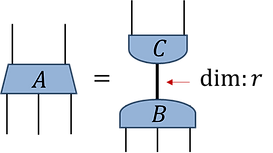These tutorials are designed to offer a practical guide on getting started with the numerical implementation of tensor network methods, focusing on how to use these methods. Accordingly, we would defer the reader interested in theoretical aspects of tensor networks, i.e. why these methods work and are useful, to one of the several excellent introductory articles from the links page

Our goal in building these tutorials was to make them accessible to anyone with a rudimentary knowledge of linear algebra, although some prior knowledge of many-body physics and of programming (in either, MATLAB, Julia or Python/Numpy) will certainly be helpful. By the completion of these tutorials you should have acquired not only the skills necessary to understand the workings of our tensor network example codes, but also to program your own codes!

Each of the tutorials should take approximately ~1hr or less to complete. The tutorials contain code snippets that we encourage readers to reproduce themselves during the course of the tutorial. Problems sets with worked solutions are also provided at the end of each tutorial, which contain both theory questions as well as programming tasks.

We kindly ask that you cite the accompanying preprint arXiv:xxxxx.xxxx if you use aspects of these tutorials in your research, in order to promote awareness of these resources. Please contact us as at "contact@tensors.net" for bug reports or suggestions for improvements.

##### Terminology:

Within these tutorials we use the following conventions:

• The order of a tensor is defined as the number of indices it has, i.e. A is an order-5 tensor.

• The rank (or decomposition rank) of a tensor w.r.t. some partition of the indices (for tensors with order > 2) specifies the minimum dimension of an exact factorization on this partition, i.e. A has rank r.

• The use of dimension will refer almost exclusively to the size of a tensor index, i.e. the number of values an index can take.• Where applicable, the text within each tutorial will refer a tensor manipulation by the MATLAB name of the routine, e.g. the operation of changing the ordering of a tensor's indices will be referred to by the MATLAB name 'permute' rather than either 'permutedims' or 'transpose' for the equivalent Julia and Python routines respectively. Of course, the accompanying code snippets can be used to infer the corresponding routine names in each of the programming languages.

##### Tutorials overview:

Tutorials 1-4 focus on the mechanics of manipulating tensors and tensor networks, including tensor contractions, decompositions, and restricted rank approximations. In order to be accessible to a broad audience, these concepts are introduced in complete generality (i.e. without reference to a specific application such as the study of quantum systems).

###### Tutorial 1: Tensor Contractions

Introduces the basics of manipulating tensors and contracting tensor networks efficiently including:

• the initialization of tensors,

• diagrammatic notation for tensors and tensor networks,

• the manipulation of tensors via 'permute' and 'reshape' functions

• binary tensor contractions and computational costs

• use of 'ncon' routine to contract networks

###### Tutorial 2: Tensor Decompositions

Introduces the basics of decomposing tensors into products of other tensors including:

• Special tensor types: diagonal, unitary, isometric tensors

• Use of singular value decomposition 'svd' to decompose tensors

• Use of spectral decomposition 'eig' to decompose tensors

• Use of QR decomposition 'qr' to decompose tensors

• The Frobenius norm and optimal restricted rank tensor truncations

###### Tutorial 3: Gauge Freedom

Introduces the basics of manipulating the gauge freedom in tensor networks and its application to network decompositions, including:

• Tree tensor networks

• Identifying the gauge freedom in tensor networks

• Creating a center of orthogonality in a tensor network

• Tensor decompositions within networks

###### Tutorial 4: Canonical Forms

Expands on the concepts, tools and applications for manipulating the gauge as introduced in the previous tutorial. Topics include:

• Multi-stage tensor decompositions

• Centers of orthogonality on tensor links

• Canonical forms in tensor networks